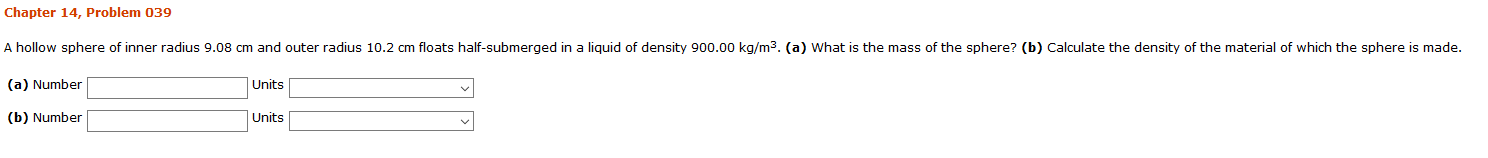# Chapter 14, Problem 039A hollow sphere of inner radius 9.08 cm and outer radius 10.2 cm floats half-submerged in a liquid of density 900.00 kg/m3. (a) What is the mass of the sphere? (b) Calculate the density of the material of which the sphere is made.(a) NumberUnits(b) NumberUnits

Question
1 viewshelp_outlineImage TranscriptioncloseChapter 14, Problem 039 A hollow sphere of inner radius 9.08 cm and outer radius 10.2 cm floats half-submerged in a liquid of density 900.00 kg/m3. (a) What is the mass of the sphere? (b) Calculate the density of the material of which the sphere is made. (a) Number Units (b) Number Units fullscreen
check_circle

Step 1

(a) Mass of the sphere is,

Step 2

(b) Density of the ...

### Want to see the full answer?

See Solution

#### Want to see this answer and more?

Solutions are written by subject experts who are available 24/7. Questions are typically answered within 1 hour.*

See Solution
*Response times may vary by subject and question.
Tagged in

### Fluid Mechanics